# Wanderlust¶

## Introduction¶

In 2015 close to 33 million Americans travelled to international regions. The following pie chart shows where people chose to travel.With little time off and so many places in the world to travel how should travelers decide where they should go?

The answer to this question becomes harder as you also include friends and family in your travel plans. When traveling in a group we are faced with many challenges. One of them is:

• Where should people travel? Each person has a list of preferences, so given this list where should they go so each of them is reasonably satisfied?

Given a list of activities that each user in the travel group would prefer to do and prior information about countries/cities for these activities, we can come up with models that successfully recommend places to visit.

A simple example of the process would be as follows:

Preferences scored out of 10 for a three person travel party are listed below:

User Activities
User A Scuba/ Snorkeling Surfing Restaurants Beer
User B History Sightseeing Beer
User C Climbing Scuba/ Snorkeling

As travel experts we have a database of countries/cities and their ratings for the above activities. A snapshot of our database is provided below:

In our database scores out of 10 are assigned to indicate the quality of an activity in a particular place.

Here is a quick look at the travel data base we will be using in this work. This data was synthetically created. We have a model for collecting this information from the internet but it is beyond the scope of this class.

In the example below, Washington would be considered better for Surfing/Sailing than Colorado.

Surfing 0 7 6 7 4 9 0 8 5 9
Restaurants 6 8 10 5 7 10 8 6 7 6
Shopping 4 6 10 2 8 10 8 6 4 4
Climbing 10 9 5 4 7 6 4 1 2 8
Snorkelling/Scuba 0 4 3 1 5 8 1 9 1 10
Hiking/Camping 9 9 6 6 7 9 4 3 4 9
Wildlife 7 7 2 4 6 8 4 6 6 8
Famous Monuments 6 7 10 2 7 7 7 4 9 4
History 5 6 10 4 10 8 2 4 8 6
Nightlife 7 8 9 3 7 9 10 9 7 5
Beer 9 6 6 9 6 8 6 6 5 4
Wine 6 4 4 6 5 10 6 6 5 3
Cost \$775 \$850 \$1500 \$450 \$950 \$2000 \$800 \$900 \$900 \$2100

By just skimming the preferences and our data, it would seem we should recommend places near the ocean famous for Beer with some History. A rough solution would look like Colorado, California and Massachusetts would be ideal locations. But is that trip affordable? Does user A prefer Surfing over Beer? These are all reasonable questions to ask when recommending the right trip.

To solve this recommendation problem we have come up with three different models each with its own distinct advantages.

The first two models are descendants of the set cover problem while the third model is a tradeoff problem we came up by ourselves. The set cover models are more biased towars making sure we find trips where each activity is equally represented. The third model which we have named as the "fun-maximizing" model lets us indulge in some activities more than others. Before we get started on the mathematics of the models, it is important to undertand how the data is laid out.

$\textbf{Data Wrangling:}$

For us to be able solve the aforementioned problem using models defined above, we require to perform some data pre processing. It will be clear why we do this when introduce the set cover model formally.

To model our problem as a set cover we need a binary data matrix. Define a matrix $A_{\text{set cover}}$ such that

$$A_{\text{set cover}}(i,j)= \begin{cases} 1 & \text{if we use activity i has a rating of 8 or greater in country j} \\ 0 & \text{otherwise} \end{cases}$$

We decided on an arbitrary threshold of 8 as a measure of quality for a given activity. Note there are cleverer ways to calculate this threshold. We could take the mean or median of every users rating for a given activity and decide on the threshold. We leave it to the user the experiment with different values for this threshold and see how the results change.

Our third model does not require us to change our data matrix in any way. Now that we've decided on data, let us talk about the mathematical models.

For the sake of notation, there are $n$ activities that the travel party is interested in and $p$ places in the world to go to.

## Mathematical Models:¶

### Model 1: The set cover and its variants¶

As mentioned in the introduction we need to compute a binary data matrix for set cover problems. It becomes clear why we need to do so once we understand how the set cover is modelled as an Integer Program.

The set cover problem is a classic problem in computer science and combinatorics and is shown to be NP-Complete.

When given a set of $\{1,2,...,m\}$ elements often known as the universe $U$ and a collection $S$ of $n$ sets whose union equals the universe, the problem is to find the smallest sub-collection of $S$ that covers the entire universe.

Most NP-complete problems can be modelled as an Integer Program.

A formal definition of the Set Cover problem as an IP is provided as follows:

$$U = \{1,2,3,4,..., m\}$$

is the universe of elements.

$$S = \{ S_1, S_2, ... , S_n\}$$

is the set of subsets containing elements of the universe.

We require that, $$\cup_{i=1}^{n} S_i = U$$

i.e the union of all subsets recovers the universe.

We define a matrix A such that, the rows correspond to elements in the universe and the columns correspond to subsets of S.

$$A_{i,j}= \begin{cases} 1 & \text{if we use S_j, and S_j contains element i in the Universe} \\ 0 & \text{otherwise} \end{cases}$$
$S_1$ $S_2$ ... $S_n$
1 1 0 0 0
2 1 0 0 0
3 1 0 0 0
... ... ... ... ...
n 0 0 1 1

$\textbf{Variable definition:}$

We define: $$x_i= \begin{cases} 1 & \text{if we use S_i in the cover} \\ 0 & \text{otherwise} \end{cases}$$

i = 1,...,n

$\textbf{Objective function}$

$$\text{argmin}_x \text{ } c^Tx$$

$\textbf{Constraint}$

$$a_i^Tx \geq 1 \text{ } \forall i=1,..,n$$

where $a_i$ represents the i'th row in A and c represents the cost vector.

The above constraint is what guarantees that for the i'th item in the universe, we have a subset $x_j$ (j=1,.., n) that covers that item.

For the basic set cover problem the cost vector is an array of ones of size n, which is equivalent to writing $\sum_{i=1}^n{x_i}$

We solve a simple set cover problem in appendix and the reader is encouraged to go through it if the above explaination is hard to follow.

#### Traveling as a set cover problem:¶

The rows of our binary data matrix correspond to activities and the columns correspond to places to visit. The (i,j) position in the matrix is set to 1 if the place offers that activity(i.e has a rating of 8 or greater). By modelling this way, we are telling our model to find the minimum number of countries required to cover every activity on the preference list. However we may not wish to find the minimum number of countries but a trip with a minimum total cost associated with it.

### Model 1.1: The Weighted Set Cover problem¶

Under the weighted set cover model, each set $S_i$ in $S$ has a weight $w_i$ associated with it i.e in the case of our problem the cost associated with traveling to i'th country.

The difference between the weighted set cover problem and set cover problem is that instead of minimizing the number of sets in $S$ that covers the universe, we find a sub collection that covers the universe and has the minimum cumulative weight.

The difference in the IP formulation is that instead of the cost vector being an array of ones, now the cost vector is the array of weights for each $S_i$ i.e cost of visiting the places in our problem.

Reference appendix  for examples of toy problems implemented as weighted set cover and set cover.

### Model 1.2: The Partial Set Cover problem¶

Often covering every element in the universe could be expensive. Given budgetary constraints, the partial set cover lets us decide how many elements in the universe we wish to cover. We could make this decision based on the available budget for travelling.

Formally :

Consider, $u_{i} \in U$ be each element of the universe. Let us define $y_{i}$ to be a binary variable that is assigned to each element $u_{i} \in U$.

$y_{i} = 1$ if and only if $u_{i}$ is not covered.

It is easy to see that if our universe has $n$ elements, and we wish to cover atmost $k$ of them, then we have atmost $n-k$ uncovered elements. Note that when $k=n$ we have the original set cover problem, i.e Model 1 and 1.1.

We define: $$x_j= \begin{cases} 1 & \text{if we use S_j in the cover} \\ 0 & \text{otherwise} \end{cases}$$

i = 1,...,p

where n := number of activities where p := number of places

Like the weighted set cover problem we wish to minimize the total cost associated with the trip.

$\textbf{Objective function}$

$$\text{argmax}_x \text{ } \sum_{j=1}^p cost(S_{j})x_j$$

$\textbf{Constraints}$

$$y_i + \sum_{j:u_{i} \in S_{j}}x_j \geq 1$$

for $i=1,...,n$.

This constraint ensures that the i'th element in the universe may not be covered by our solution. If a $y_i =1$, it would mean we can ignore element $u_i$

$$\sum_{i=1}^n y_i \leq n-k$$

.

This constraints makes sure that we cover atmost k elements out of the n possible elements of the universe. $$x_j \geq 0$$ for $j=1,....,p$. $$y_i \geq 0$$ for $i=1,....,n$.

### Model 1.2.1: Mild variant to the Partial Set Cover problem¶

The partial set cover problem guarantees that we pursue k out m activities but it does not guarantee that any specific activity would be covered by our trip. We came up with mild variant that would help us build this feature into our model.

We introduce a parameter $\lambda_i$ for each $u_i \in U$. By setting $\lambda_i = 0$ we make sure that activity $i$ is covered in the $k$ out of the $n$ activities we agree to cover. So our constraint now looks like,

$\textbf{Constraints}$

$$y_i + \lambda_i+\sum_{j:u_{i} \in S_{j}}x_j \geq 1$$

for $i=1,...,n$.

Introducing $lambda_i$ converts the partial set cover problem of covering at most $k$ elements to one, where in addition to covering $k$ elements we wish to cover element $u_i$ in the universe.

Depending on the cost associated with $u_i$, we see that, $u_i$ is either a part of the $k$ elements covered, or $u_i$ is covered in addition to the $k$ elements.

### Model 2: Fun Maximizing Model¶

So far we have considered situations in which our primary goal is either a) to cover all activities in the universe in the most efficient way (weighted set cover problem) or b)to cover at most $k$ out of the $n$ activities of the universe.

Our focus now is to develop a model that can help travellers maximize the quality of their activities while balancing it against the cost of the trip. For example, if the group likes surfing a whole lot more than everything else, they might be more willing to give up other things at the cost of being able to Surf in two separate locations. We no longer try and cover every activity, on the contrary we try to maximize the total amount of fun they can have on the trip.

A formal mathematical model for this problem is,

Define: $$x_i= \begin{cases} 1 & \text{if we use S_i in the cover} \\ 0 & \text{otherwise} \end{cases}$$

i = 1,...,p

where n := number of activities where p := number of places

$\textbf{Objective function}$

$$\text{argmax}_x \text{ } \sum_{j=1}^n \lambda_j \sum_{i=1}^p a_{ji}x_i$$

$\textbf{Constraints}$

$$c^Tx \leq budget \text{ }$$

where $a_ij$ represents the (i,j)th cell in A and c represents the cost vector.

$\textbf{Intuition}$

To briefly understand this model, fix activity $j$ to be surfing. The innermost sum corresponds to the total quality of your surfing experience. Maximizing the sum:= $\sum_{i=1}^p a_{ji}x_i$ sums over the quality of surfing across all places that offer surfing. Note that as long as the optimal solution satisfies that budget constraint, under this model, the optimal solution would pick the best place for surfing within that budget.

Each $\lambda_j$ represents the importance of activity $j$. Now say $\lambda_{\text{surfing}}=100$ while $\lambda_j$ for all other activities was equal to 1. The model interprets this as saying that surfing is an activity that is unanimously enjoyed in the group. In this case, our model strives to pick the best place for surfing while ignoring some other activities.

Note that unlike the full set cover model, this model does not provide any guarantees that all needs of every user will be fulfilled. Instead it looks at the groups' needs as a whole and tries to maximize the total amount of fun that can be had.

## GLOBAL VARIABLES¶

Note to User: Run Helper Functions before proceeding with codes for the mathematical models

In :
#-------------------------------------------------------------
# Load data for all models
# A_set_cover : Binary data matrix for set cover models
# A : the raw data
# cost : The cost of going to each country
#------------------------------------------------------------
A_set_cover, A, cost, places, activities = generate_data("BiswasKameswaraSarma_data.csv");
(n,p) = size(A)

#-----------------------------------------------------------
# Visualize our data matrix for
# binary matrix := A_set_cover
# data matrix   := A
#-----------------------------------------------------------

#-----------------------------------------------------------
#   USER Preference to visualize raw data or set cover data
#-----------------------------------------------------------
# d = A
d = A_set_cover

B = visualize_data(d, places, activities);

println("Visit for another visualization of data: http://abiswas3.github.io/places_to_go.html")

Row view:
0 0 0 0 0 1 0 1 0 1 Surfing/ Sailing
0 1 1 0 0 1 1 0 0 0 Restaurants
0 0 1 0 1 1 1 0 0 0 Shopping
1 1 0 0 0 0 0 0 0 1 Climbing
0 0 0 0 0 1 0 1 0 1 Snorkelling/ Scuba
1 1 0 0 0 1 0 0 0 1 Hiking/Camping
0 0 0 0 0 1 0 0 0 1 Wildlife
0 0 1 0 0 0 0 0 1 0 Famous monuments
0 0 1 0 1 1 0 0 1 0 History
0 1 1 0 0 1 1 1 0 0 Night life
1 0 0 1 0 1 0 0 0 0 Beer
0 0 0 0 0 1 0 0 0 0 Wine

Column view with costs for each country:
0 0 0 1 0 1 0 0 0 0 1 0 Colorado $775.0 0 1 0 1 0 1 0 0 0 1 0 0 Washington$850.0
0 1 1 0 0 0 0 1 1 1 0 0 New York $1500.0 0 0 0 0 0 0 0 0 0 0 1 0 Wisconsin$450.0
0 0 1 0 0 0 0 0 1 0 0 0 Texas $950.0 1 1 1 0 1 1 1 0 1 1 1 1 California$2000.0
0 1 1 0 0 0 0 0 0 1 0 0 Nevada $800.0 1 0 0 0 1 0 0 0 0 1 0 0 Florida$900.0
0 0 0 0 0 0 0 1 1 0 0 0 Massachusets $900.0 1 0 0 1 1 1 1 0 0 0 0 0 Hawaii$2100.0

Most popular activity amongst all state: Night life
Visit for another visualization of data: http://abiswas3.github.io/places_to_go.html


$\textbf{Julia Implementation for Models 1 and 1.1 :}$

In [ ]:
# Model 1.1: The the weighted set cover as an ILP
using JuMP

function weighted_set_cover()
m = Model()
@variable( m, 0 <= x[1:p] <= 1, Int) # p countries

# Constraint to make sure every element in the
# universe is covered
@constraint(m, A_set_cover[:,:]*x .>= 1)

# Minimize the total cost of the trip
@objective(m, Min,  dot(cost,x))
solve(m)

return getvalue(x), dot(cost,getvalue(x))
end
;


$\textbf{Julia Implementation for Model 1.2 :}$

In [ ]:
# Model 1.2: Partial set cover (Travel Database)
using JuMP,PyPlot

function partial_set_cover(lambda, k)
m = Model()
@variable( m, x[1:p],Bin) # which places we visit
@variable(m, y[1:n],Bin) # which activities are covered

for i=1:n
#this constraint considers each activity as a candidate for the k possible activities
@constraint(m, y[i]*lambda[i]+ sum{A_set_cover[i,j]*x[j], j=1:p} >=1)
end

@constraint(m, sum(y) <= n-k) #this constraint ensures atmost k activities are covered
@constraint(m, x.>=0) #this constraint sets the lower bound on x
@constraint(m, y.>=0) #this constraint sets the lower bound on y
@objective(m, Min, dot(cost,x))
status=solve(m)

return getvalue(x), getvalue(y)
end
;

# Solved with data in the Analysis and discussion section


$\textbf{Julia Implementation for Model 2:}$

In :
# Model 2: Fun Maximizing model
function solve_fun_maximizing_model(lambda, budget)

m = Model()
@variable( m, 0 <= x[1:p] <= 1, Int)
@constraint(m, dot(cost,x) <= budget) #this constraint ensures we satisfy a given budget
@objective(m, Max,  sum{lambda[j]*sum{A[j,i]*x[i], i=1:p}, j=1:n})
solve(m)

return getvalue(x), dot(cost, getvalue(x)) ;
end
;
# Solved with data in the Analysis and discussion section


# Comparison of models¶

There is not much to analyse about the weighted set cover model. The model finds us the cheapest trip that guarantees we cover every single activity specified by the group.

However this model has certain disdavantages:

• What if the cheapest trip that covers every element turns out to be too expensive for certain people in the group? Do we drop those people from the travel party or do we drop some activities? In that case which activities should be dropped?

These questions are better answered by the Partial set cover model. The plot and section of code illustrates this concept. On the x axis we have number of activities covered while on the y axis we plot, the cost of the trip.

From the picture below and the weighted set cover problem it is clear that the cost of covering all items is $\$4275$. It is interesting to note that if we only had \$3000, we would still be able to cover 11 out of the 12 items in the universe.

Thus if we were willing to do away with just one activity we would save \$1375, as long as we are willing to sacrifice viewing Famous Monuments. In : #-------------------------------------------------- # WEIGHTED SET COVER SOLUTION #-------------------------------------------------- x, price = weighted_set_cover() view_trip_details(x, n, p, price) mapSolution(x)  Cost of trip: 3675.0 Places to go: Colorado California Massachusets Items not covered: None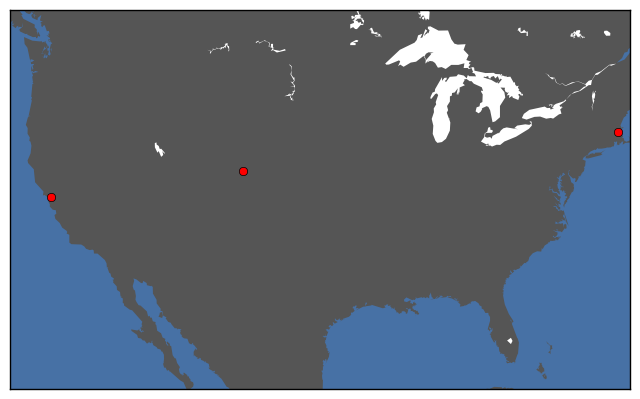In : # Partial Set cover(Model 1.2) with no manadatory activities lambda=ones(n,1); lambda = 1 #Surfing/ Sailing lambda = 1 #Restaurants lambda = 1 #Shopping lambda = 1 #Climbing lambda = 1 #Snorkelling/ Scuba lambda = 1 #Hiking/Camping lambda = 1 #Wildlife lambda = 1 #Famous monuments lambda = 1 #History lambda= 1 #Night life lambda= 1 #Beer lambda= 1 #Wine opt_cost_vector_partial_set_cover, xiter, yiter = partial_set_cover_all(lambda, cost); plot_cost_vs_k(opt_cost_vector_partial_set_cover) # ----------------------------------------------------------------------- # Parameters: # User can change variable number_of_items_covered to view which # activities are covered number_of_items_covered = 11 # ------------------------------------------------------------------------ view_trip_details(xiter[number_of_items_covered], n, p, opt_cost_vector_partial_set_cover[number_of_items_covered]);  Cost of trip: 2775.0 Places to go: Colorado California Items not covered: Famous monuments,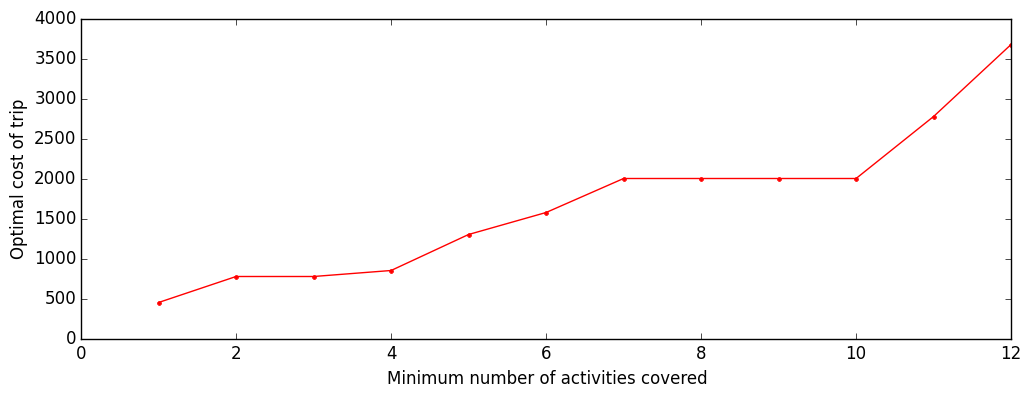The plot points out interesting features about the preferences of the user. If the users were willing to sacrifice just 2 of out the 12 activities it would seem like they could get a large cost benefit. In : mapSolution(xiter[number_of_items_covered])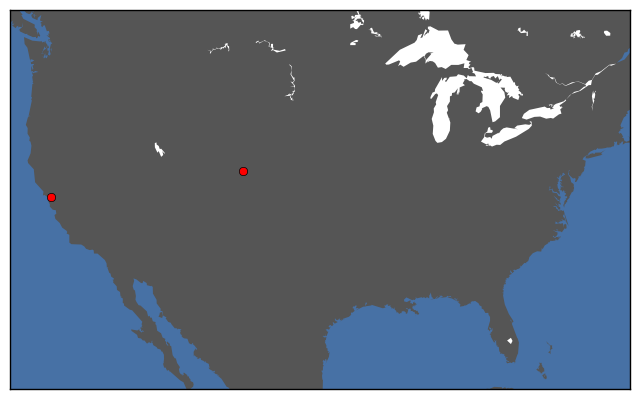But what if the travel group really liked Famous Monuments? To solve this problem we tell our partial set cover model, that Famous Monuments is a mandatory activity. The reader is encouraged to change the mandatory activity and see how the cost plot and recommended trip changes. In : #Partial set cover(Model 1.2) with a mandatory activity lambda=ones(n,1); lambda = 1 #Surfing/ Sailing lambda = 1 #Restaurants lambda = 1 #Shopping lambda = 1 #Climbing lambda = 1 #Snorkelling/ Scuba lambda = 1 #Hiking/Camping lambda = 1 #Wildlife lambda = 0 #Famous monuments - MANDATORY !!!!!!!!!!!!!!!!!!! lambda = 1 #History lambda= 1 #Night life lambda= 1 #Beer lambda= 1 #Wine opt_cost_vector_partial_set_cover, xiter, yiter = partial_set_cover_all(lambda, cost); plot_cost_vs_k(opt_cost_vector_partial_set_cover) # ----------------------------------------------------------------------- # Parameters: # User can change variable number_of_items_covered view which # activities are covered number_of_items_covered = 11 # ------------------------------------------------------------------------ view_trip_details(xiter[number_of_items_covered], n, p, opt_cost_vector_partial_set_cover[number_of_items_covered]);  Cost of trip: 2900.0 Places to go: California Massachusets Items not covered: Climbing,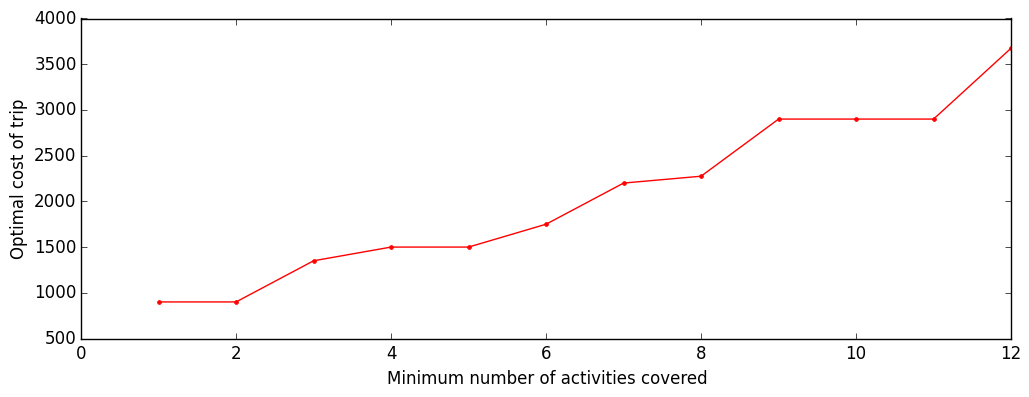In : mapSolution(xiter[number_of_items_covered])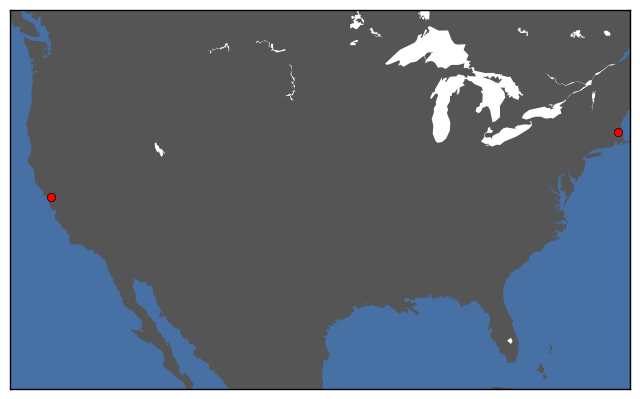Now at the same cost we view Famous Monuments but sacrifice Climbing. The user may feel free to play around with the parameters which denote preferences and see how their trip changes. Based on the plot the group may decide to abandon certain activities or certain users that bring costly activites. It would depend on the preference of the user. So we've brought in a tradeoff between cost and activities but even the partial set cover model has major drawbacks. Since the setcover models require us to have a binary data matrix we lose a large amount of information when we binarize our data matrix. For example, Washington has a 7 for Famous Monuments and Wisconsin has a rating of 2. Our set cover model thresholds anything above 7 as a 1. So according to our set cover model Wisconsin and Washington have the same rating for Famous Monuments but that's clearly not true. If the traveler could he'd be better off in Washington than Wisconsin if he had a small inclination towards monuments as well. Another interesting thing to note is the preference model we have set up. Making Famous Monuments mandatory ensured we visit a place that covered it. What if we cared more about Famous Monuments than we cared about any other activity? Then we'd probably like to go to all the places with good monuments and fill in the rest of our trip with whatever remains based on some preference model. This is where our last model does really well. The fun maximizing model tries to maximize the total amount of fun that can be had. So if we claim that we really cared about monuments, it would give us an itenary heavily biased towards Famous Monuments. A drawback of this model is however we are no longer guaranteed to cover all activities. The fun maximizing activity has one constraint and that is to remain within a specified budget. For a given budget, we wish to maximize the total amount of fun that we can have. Let's take a look at the model in action to get a better understanding Assume we have a budget of \$4275 to begin with and we are indifferent about all activities. How does our Fun Maximizing model perform?

In :
# Fun Maximizing model(Model 2)
# Parameters: equal preference for all activites in the Universe

#------------ Model Preferences----------------------------------------
# User may change the following parameters to see how the trip changes:
#----------------------------------------------------------------------
lambda = ones(n)
# Currently all activities have equal preferences

lambda = 1 #Surfing/ Sailing
lambda = 1 #Restaurants
lambda = 1 #Shopping
lambda = 1 #Climbing
lambda = 1 #Snorkelling/ Scuba
lambda = 1 #Hiking/Camping
lambda = 1 #Wildlife
lambda = 1 #Famous monuments
lambda = 1 #History
lambda= 1 #Night life
lambda= 1 #Beer
lambda= 1 #Wine

# Budget for trip
budget = 4275; # currently set at the same cost as weighted set cover

x, price = solve_fun_maximizing_model(lambda, budget)
view_trip_details(x, n, p, price);
mapSolution(x)
#-----------------------------------------------------------------------

Cost of trip: 4275.0

Places to go:
Washington
Texas
Florida

Items not covered:
Wildlife, Famous monuments, Wine,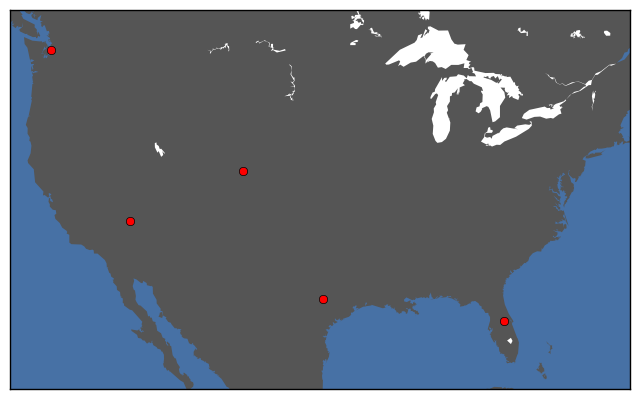The fun maximizing model fails to cover 3 activites but also lets us travel to 2 more places than the weighted set cover model.

While this may seem far inferior at first sight, this model is really leveraging the fact that Washington has a Famous Monuments rating of 7, Nevada a Wine rating of 6 and Colorado a Wildlife rating of 7.

This would mean that our set cover model wouldn't include these places as sources for the above activites because of it's binary nature but they still do have a lot to offer which is lost by the set cover models.

The real advantage of the Fun Maximizing model can be understood when we bring in preferences.

We will look at two cases of preferences. The user is encouraged to play with the preference parameters and see what kind of trip shows up.

### CASE I:¶

Say our travel group absolutely loves famous monuments, as discussed before. The ideal trip would involve visiting places with extremely high ratings for monuments.

Let's change some of the parameters to see what happens.

In :
# Model 2, CASE I:
# Parameters: equal preference for all activites in the Universe

#------------ Model Preferences----------------------------------------
# User may change the following parameters to see how the trip changes:
#----------------------------------------------------------------------
lambda = ones(n)
# Currently all activities have equal preferences, (scored out of 100)
#except Famous monuments

lambda = 1 #Surfing/ Sailing
lambda = 1 #Restaurants
lambda = 1 #Shopping
lambda = 1 #Climbing
lambda = 1 #Snorkelling/ Scuba
lambda = 1 #Hiking/Camping
lambda = 1 #Wildlife
lambda = 100 #Famous monuments <------- THEY REALLY LIKE FAMOUS MONUMENTS
lambda = 1 #History
lambda= 1 #Night life
lambda= 1 #Beer
lambda= 1 #Wine

# Budget for trip
budget = 4275; # same as earlier
#-----------------------------------------------------------------------

x, price = solve_fun_maximizing_model(lambda, budget)
view_trip_details(x, n, p, price);
mapSolution(x)

Cost of trip: 4275.0

Places to go:
Washington
Texas
Massachusets

Items not covered:
Surfing/ Sailing, Snorkelling/ Scuba, Wildlife, Wine,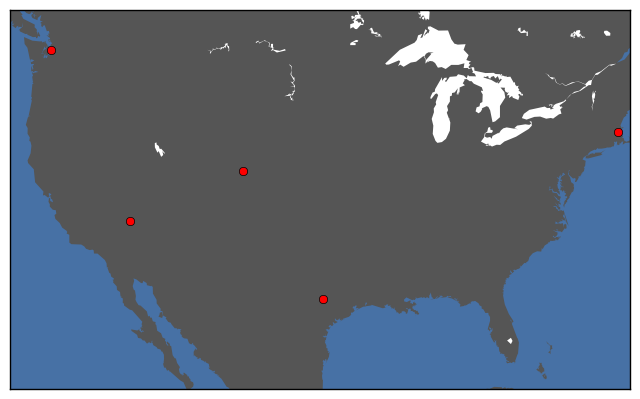But why does New York not show up? It has the best Famous Monuments. The answer to that question is our budget constraint. Currently, given the budget of \$4275, it is still more profitable to just go to Massachusetts and these other states than to visit New York and fewer states. (Note: All activities still have a preference of 1, which while small still adds up) Two ways to remedy this would be to 0 out the preferences of some other activities or increase the budget. We'll leave the tinkering of preferences to the user and demonstrate what increasing the budget does. In : # Budget for trip budget = 5000; # Increased budget x, price = solve_fun_maximizing_model(lambda, budget) view_trip_details(x, n, p, price); mapSolution(x)  Cost of trip: 5000.0 Places to go: Washington New York Texas Nevada Massachusets Items not covered: Surfing/ Sailing, Snorkelling/ Scuba, Wildlife, Beer, Wine,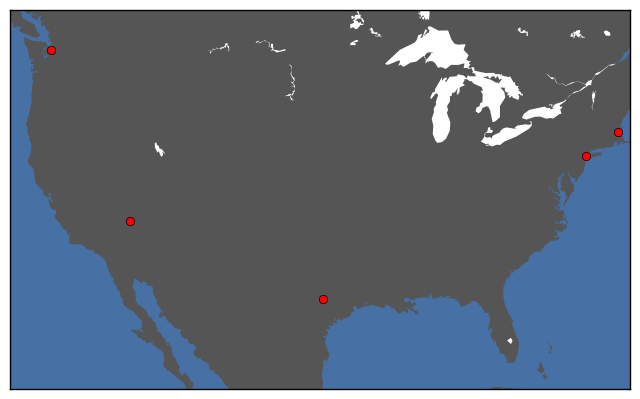As expected, New York and Massachusetts both make the trip and the trip is heavily biased towards Famous Monuments. ### CASE II:¶ The fun maximizing model also incorporates negative preferences. A good example of where this comes in handy is the following example. Let's assume that the same travel party that really liked Famous monuments, has a member Charlie, who recently broke his foot. Charlie is an avid sailer and cannot stand watching others surf and sail while sitting out. The group wants to avoid placecs that offer good Surfing or Sailing. They give negative preferences to surfing while still wishing to view Famous Monuments. In : # Model 2, CASE II: # Parameters: equal preference for all activites in the Universe #------------ Model Preferences---------------------------------------- # User may change the following parameters to see how the trip changes: #---------------------------------------------------------------------- lambda = ones(n) # Currently all activities have equal preferences, (scored out of 100) lambda = -100 #Surfing/ Sailing <--------- CHARLIE DOESN'T WANT TO SURF lambda = 1 #Restaurants lambda = 1 #Shopping lambda = 1 #Climbing lambda = 1 #Snorkelling/ Scuba lambda = 1 #Hiking/Camping lambda = 1 #Wildlife lambda = 100 #Famous monuments lambda = 1 #History lambda= 1 #Night life lambda= 1 #Beer lambda= 1 #Wine # Budget for trip budget = 4275; # currently set at the same cost as weighted set cover x, price = solve_fun_maximizing_model(lambda, budget) view_trip_details(x, n, p, price); mapSolution(x) #-----------------------------------------------------------------------  Cost of trip: 3975.0 Places to go: Colorado New York Nevada Massachusets Items not covered: Surfing/ Sailing, Snorkelling/ Scuba, Wildlife, Wine,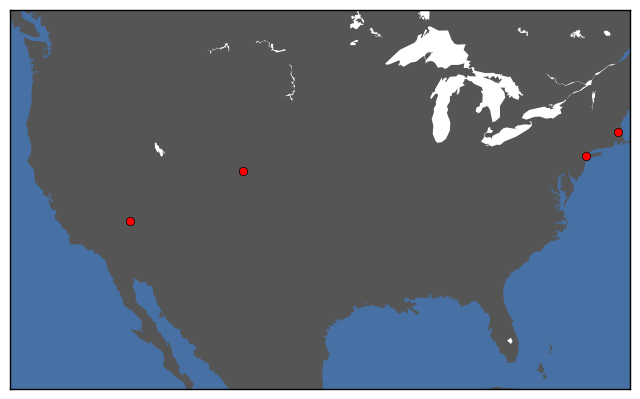As expected we visit New York and Massachusetts. But we now visit Colorado and Nevada which have ratings of 0 for Surfing and Sailing. So we've avoided surfing while seeing Monuments too. Unlike the covering models we have avoided a large number of activities while trying to achieve this. We encourage to user to play around with the preferences. What would happen if the group just gave Charlie a huge preference to drink his sorrows away? How would you model the preferences? # Helper Functions¶ In [ ]: Pkg.add("PyPlot") using NamedArrays using PyPlot ########################################################## # Function that calculates partial set cover objective # under the partial set cover model # Arguments: # lambda: lambda[i] = 0 if i'th activity is mandatory # lambda[i] = 1 otherwise # # cost: cost associated with each activity # # Outputs: # opt_cost_vector_partial_set_cover: optimal cost vector # for covering k activities # xiter: Places covered by k activities. # yiter: List of which k activities are covered in # optimal solution. ########################################################## function partial_set_cover_all(lambda, cost) # array to store cost of covering items # opt_cost_vector_partial_set_cover[i] = cost of covering i items in the universe opt_cost_vector_partial_set_cover = zeros(n,1); xiter=[] yiter=[] for iter = 1:n x, y = partial_set_cover(lambda, iter) opt_cost_vector_partial_set_cover[iter]=dot(cost, x); push!(xiter, x) push!(yiter, y) end return opt_cost_vector_partial_set_cover, xiter, yiter end ; ########################################################### # Function that generates database from a .csv file # Arguments: datafile to be read # # Outputs: # A_set_cover: Binary data matrix for set cover problems # A : Data matrix for Fun maximization model # cost : Cost vector associated with the places in # database # places : vector of places in the universe # activities : vector of activities that possible to explore ############################################################ function generate_data(filename) #----------------------------------------- # A .csv file that contains our raw A matrix #----------------------------------------- raw = readdlm(filename,','); (n,p) = size(raw) n_places = 2:p n_activities = 2:n places = raw[1,n_places][:] activities = raw[n_activities - 1,1][:] cost = raw[n, n_places][:] data = NamedArray(raw[n_activities - 1,n_places], (activities,places), ("places","activities") ); #----------------------------------------- # Binarizing our raw A matrix, with a threshold of 7 # And constructing a cost vector. For the sake of convenience, # each column was summed previously in the data and we did not # have to do it explicitly #----------------------------------------- A = zeros(Int64, n-2, p-1) A_set_cover = zeros(Int64, n-2, p-1) cost = zeros(Float64, p-1) for( i in 2:p) cost[i-1] = raw[n,i] end for (i=2:n-1) for(j= 1:p-1) if data[i,j] > 7 A_set_cover[i-1, j] = 1 end A[i-1,j] = data[i,j] end end return A_set_cover, A, cost, places, activities end ; ########################################################## # Function that helps visualize the database matrix # Arguments: # d: Binary data matrix for set cover models # places: vector of places obtained from database # activities: vector of activities obtained from database # Outputs: # Tabular representation of activities, places and ratings ########################################################## function visualize_data(d, places, activities) B = 0 # activity that is common to most countries. c = 1 println("Row view:") for i=1:n for j=1:p print(d[i,j]," ") end println(activities[c+1]," ") c +=1 end println() println("Column view with costs for each country:") c = 1 for i=1:p for j=1:n print(d[j,i]," ") end println(places[c]," \$", cost[c])
c +=1
end
println()

c = 1
pos = 1
max = 1
for i=1:n
count = 0
for j=1:p
if (d[i,j] == 1)
count +=1
end
end
c +=1
if count > max
max = count
pos = c-1
end
#     println(activities[c]," :", count)
end
println()
println("Most popular activity amongst all state: ", activities[pos+1])
B = max;

return B
end
;

##########################################################
# Function that plots trip details
# Arguments:
#   x : x[i] = 1 if i'th place in the trip
#       x[i] = 0 otherwise
#
#   n : number of activities
#   p : number of places
#   cost : cost of trip
#  Outputs: Places covered in trip
##########################################################
function view_trip_details(x, n, p, cost)
println("Cost of trip: ", cost)
println()
println("Places to go: ")
acts = zeros(n)
for i=1:p
# If the place is in the trip
if x[i] > 0
println(places[i])
for j=1:n
# activity has been covered
if A_set_cover[j, i] > 0
acts[j] = 1
end
end
end
end

println()
count = 0
println("Items not covered: ")
for i=1:n
if acts[i] == 0
print(activities[i+1], ", ")
count +=1
end
end

if count == 0
println("None")
end

end
;
##########################################################
# Function that plots optimal cost Vs number of
# activities for the partial set cover problem
# Arguments:
#   opt_cost_vector_partial_set_cover: Output from
#   the partial set cover model. Output contains a
#   vector that shows the cost associated for k
#   activities. Users can explore values of k from
#   1 to n, where n=|U|.
#
# Outputs: Plot showing optimal cost Vs activities
##########################################################

function plot_cost_vs_k(opt_cost_vector_partial_set_cover)

figure(figsize=(12,4))
plot(1:n,opt_cost_vector_partial_set_cover,".-r")
xlabel("Minimum number of activities covered")
ylabel("Optimal cost of trip")

end
;

using PyPlot
using PyCall
@pyimport mpl_toolkits.basemap as basemap

##########################################################
# Function that plots paces we visit
#
# Arguments:
#   places_to_visit: Vector with places to visit
#
# Outputs: Map of USA showing where we go
##########################################################
function mapSolution(places_to_visit)

cities_all = [:DEN, :SEA, :JFK, :MSN, :AUS, :LAX, :LAS, :MCO, :BOS, :HNL]

p = 0
for i=1:size(places_to_visit)
if places_to_visit[i] > 0
p += 1
end
end

data = zeros(p,2)
cities = []

d =
[
39.7392 -104.9902
47.6062 -122.3320
40.7127 -74.0059
43.0730 -89.4012
30.2671 -97.7430
37.8715 -122.2727
36.1699 -115.1398
28.5383 -81.3792
42.3600 -71.0588
39.7463 -84.2575
]

count = 1
for i=1:size(places_to_visit)
if places_to_visit[i] > 0
data[count,1] = d[i,1]
data[count,2] = d[i,2]
push!(cities, cities_all[i])
count +=1
end
end

lat = Dict(zip(cities,data[:,1]))
lon = Dict(zip(cities,data[:,2]))

m=basemap.Basemap(projection="merc", resolution="l",llcrnrlat=23,llcrnrlon=-126,urcrnrlat=50,urcrnrlon=-70)
m[:drawmapboundary](fill_color="#4771a5")
m[:fillcontinents](color="#555555")

#     plot airports
for i in cities
m[:plot](lon[i], lat[i], "ro" ,latlon=true)
end

end
;


# Conclusion¶

It should be noted that each model has its own advantages and disadvantages. The weighted set cover model guarantees every activity is covered at the cost of not having budgetary constraints. The partial set cover model helps us cover certain activities while controlling the price of the trip. However it does not let the travelers truly indulge in one specific kind of activity over another. At it's heart it is still a covering problem. The fun maximizing problem is the most flexible model, which lets us define a budget and an elborate preference list but it does not guarantee of any activity being covered. It biases the solutions towards the preferences of the users as a whole.

It would depend on the user which model suits his travel needs better. There is no one clearly better model.

It should be noted that Integer programs do not scale well. As the number of places and activities increase we may have to come up with approximate solutions to our models. One possible approximation is provided in Appendix. We demostrate that solving this instance of the problem as an LP gives us the same solution.

$\textbf{Appendix 1}$:

• It is well known that Integer programs do not scale well. Everything seems well and good with a dataset of 8-12 countries and activities. However there are well over 1000 cities in the world and the list of preferences of travelers is much more expansive in practice than what we have demonstrated. What can be done if we were working with lots and lots of data?

All Integer programs can be written as Linear programs. And we know linear programs can be solved extremely quickly with even millions of variables.

We can just round the solution of the linear program and get integer solutions.

The question is does this give us accurate enough solutions? It can be shown that there is a deterministic rounding procedure does pretty well. Let's take a look at the analysis:

##### Approximate it as an LP:¶

We formulate the same problem as above, with the difference being, we do not have an integer constraint on the variables.

Let U = $\{e_1, e_2,.., e_j, ..., e_k\}$ be the universe of elments

Let S = $\{s_1, s_2,.., s_i, ..., s_m\}$ be the set of sets which contain the above elements.

If $x_i = 1$ then set $s_i$ is in the cover C

Let $y$ be the solution the linear program program.

We Define:

$x_i$ = 1 if $y_i \geq \frac{1}{B}$

$x_i$ = 0 otherwise

where B is the most popular element in the universe

Formally : $B_j$ = number time $e_j$ is present in S,

then $B = max B_j$ for j=1,..,k

$\textbf{Theorem}$ : The above deterministic algorithm that rounds $x_{LP}$ to integer values x so that the objective value Z(x) $\leq$ B${OPT}_{IP}$, where B is the maximum number of sets that any element e_j occurs in. So this gives a B-approximation of set cover.

$\textbf{Proof}$ :

We would need to show two things to complete the proof. First we would have to show that this strategy does indeed produce a legitimate cover and then we would like to prove the bound mentioned in the theorem.

The constraints of the linear program gives us:

$\sum_{j=1}^m a_{ij}y_j \geq 1$ for all i= 1,...k.

Let's pick the i'th item in the Universe.

Thus there must exist some $y_j$ for j =1,...,m that is greater than $\frac{1}{B_i}$, which would make it greater than $\frac{1}{B}$

Note: If the above claim were false we would have $y_j < \frac{1}{B_i}$ for all j=1,...,m . If that were true $\sum_{j=1}^m a_{ij}y_j$ could never be greater than equal to one; and we would have violated one of our constraints.

Since i was general this would apply to all items in the universe, thus for each item we would have at least one $y_j \geq \frac{1}{B}$ which would make the corresponding $x_j$ equal to 1 and we would have covered that element.

This concludes the proof to show that this strategy does indeed produce a set cover.

Now we move onto the proof which bounds the error:

Let Z(x) be the objective value of the problem where x is the solution using the rounding strategy.

We know that when

If $x_i = 1$ then $y_i > \frac{1}{B}$ where y is the solution to the linear program for all i=1,...,m

Thus :

$y_iB \geq 1$

$$Z(x) = \sum_{i=1}^m x_i \leq \sum_{i=1}^m x_i(y_i)B$$

We know that $x_i$ can be at most 1

thus we would get

$$Z(x) = \sum_{i=1}^m x_i \leq B\sum_{i=1}^m y_i$$$$Z(x) = \sum_{i=1}^m x_i \leq B*OPT_{LP}$$

we know that any $OPT_{LP} \leq OPT_{IP}$

$$\therefore Z(x) \leq B*OPT_{IP}$$

which concludes are proof.

Thus if the value for B is small, we can get very close to the real solution and still scale upto thousands and even million places and activities.

##### Greedy Approximation:¶

There is a greedy algorithm that gets to with log(n) times the optimal solution where n is related to the number of plaes and activities. Since this is not an algorithms class, we've decided that the proof of this analysis is beyond the scope of this class.

For the interested reader please refer to Greedy Approximation of set cover

In :
# Model 1  LP approximation code
using JuMP

m = Model()
@variable( m, 0 <= y[1:p] <= 1)
@constraint(m, A_set_cover[:,:]*y .>= 1)
@objective(m, Min,  dot(cost,y))
solve(m)

opt_lp = getobjectivevalue(m)

# the rounding conversion
x = zeros(Int64, p)
c = 1

# rounding the LP solution
for i in getvalue(y)
if i > 1/B
x[c] = 1
end
c +=1
end

println()
println("B =",B)

# Note in the case of our problem, the solution of the Linear
# program approximation was exactly the same as the Integer program
# solution. The worst case scenerio could be terrible, as shown above,
# in practice this method is pretty useful.
view_trip_details(x, n, p,dot(cost, x) )
mapSolution(x)
println("Exact same solution as the weighted set cover with a LP")

B =5
Cost of trip: 3675.0

Places to go:
California
Massachusets

Items not covered:
None
Exact same solution as the weighted set cover with a LP$\textbf{Appendix 2}$: $\textbf{Toy Problems}$

In [ ]:
# Model 1, set cover (Toy Example)
#-----------------------------------------
# Data matrix
#-----------------------------------------
A = [
1 0 0 0 ; # 1
1 0 0 0 ; # 2
1 0 0 0 ; # 3
0 1 0 1 ; # 4
0 0 1 1 ; # 5
]

n = 4 # number of sets
M = 5 # Universe

#-----------------------------------------
# Cost vector
#-----------------------------------------
cost = [1;1;1;1]

using JuMP

m = Model()
@variable( m, x[1:n], Bin)
@constraint(m, A[:,:]*x .>= 1)
@objective(m, Min,  dot(cost,x))
solve(m)

#-----------------------------------------
# Display the output of the program
#-----------------------------------------
c = 1
println("Sets used in the cover")
for i in getvalue(x)
if (i == 1)
println("S_",c)
end

c +=1
end
println()
println("The number of sets used from the collection : ",getobjectivevalue(m))

In [ ]:
# Model 1.1 weighted set cover (Toy Example)
#-----------------------------------------
# Data matrix
#-----------------------------------------
A = [
1 0 0 0 ; # 1
1 0 0 0 ; # 2
1 0 0 0 ; # 3
0 1 0 1 ; # 4
0 0 1 1 ; # 5
]

n = 4 # number of sets
M = 5 # Universe

#-----------------------------------------
# Cost vector
#-----------------------------------------
cost = [100;24;25;50]

using JuMP

m = Model()
@variable( m, x[1:n], Bin)
@constraint(m, A[:,:]*x .>= 1)
@objective(m, Min,  dot(cost,x))
solve(m)

#-----------------------------------------
# Display the output of the program
#-----------------------------------------
c = 1
println("Sets used in the cover")
for i in getvalue(x)
if (i == 1)
println("S_",c)
end

c +=1
end
println()
println("The number of sets used from the collection : ",getobjectivevalue(m))

In :
#Model 1.2 partial set cover (Toy Example)
#define k, k is the most number of activities that need to be covered.

k=3
#-----------------------------------------
# Data matrix
#-----------------------------------------
A = [
1 0 0 0 ; # 1
1 0 0 0 ; # 2
1 0 0 0 ; # 3
0 1 0 1 ; # 4
0 0 1 1 ; # 5
]

n = 4 # number of sets
M = 5 # Universe

#-----------------------------------------
# Cost vector
#-----------------------------------------
cost = [1;1;1;1]
lambda= ones(5,1);
lambda=0;

using JuMP
m=Model()
@variable(m, x[1:n],Bin)
@variable(m, y[1:M],Bin)

for i=1:M
@constraint(m, y[i]*lambda[i]+ sum{A[i,j]*x[j], j=1:4} >=1)
end
@constraint(m, sum(y) <= M-k)
@constraint(m, x.>=0)
@constraint(m, y.>=0)
@objective(m, Min, dot(cost,x))
status=solve(m)

println(getvalue(x))
println(getvalue(y))

[1.0,1.0,0.0,0.0]
[0.0,0.0,0.0,0.0,1.0]